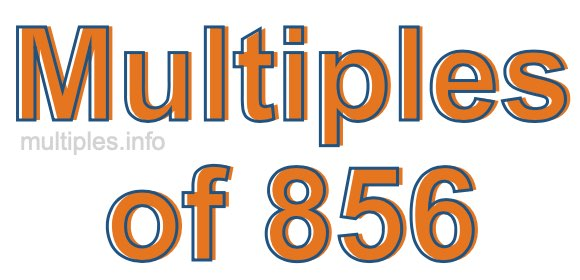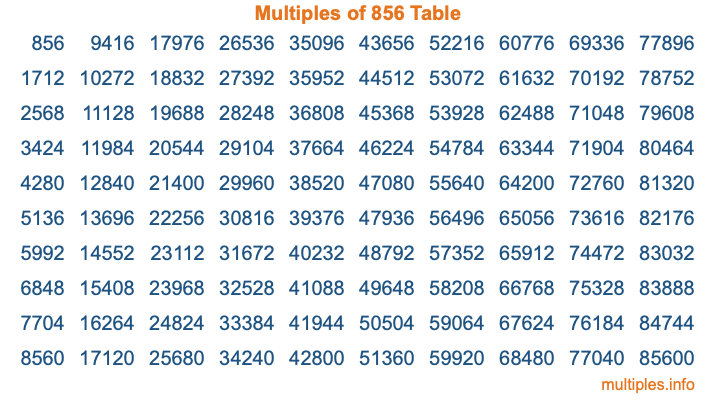Multiples of 856Welcome to the Multiples of 856 page. Here we will first teach you everything you will ever need to know about the multiples of 856, and then give you a study guide summary of everything we taught you to make sure you remember it all. Use this page to look up facts and learn information about the multiples of 856. This page will make you a multiples of eight hundred fifty-six expert!

Definition of Multiples of 856
Multiples of 856 are all the numbers that when divided by 856 equal an integer. Each of the multiples of 856 are called a multiple. A multiple of 856 is created by multiplying 856 by an integer.

Therefore, to create a list of multiples of 856, you start with 1 multiplied by 856, then 2 multiplied by 856, then 3 multiplied by 856, and so on for as long as you want. Thus, the list of the first five multiples of 856 is 856, 1712, 2568, 3424, and 4280. To see a larger list of multiples of 856, see the printable image of Multiples of 856 further down on this page. We also have a category where you can choose any nth multiple of 856.

Multiples of 856 Checker
The Multiples of 856 Checker below checks to see if any number of your choice is a multiple of 856. In other words, it checks to see if there is any number (integer) that when multiplied by 856 will equal your number. To do that, we divide your number by 856. If the the quotient is an integer, then your number is a multiple of 856.

Is  a multiple of 856?

Least Common Multiple of 856 and ...
A Least Common Multiple (LCM) is the lowest multiple that two or more numbers have in common. This is also called the smallest common multiple or lowest common multiple and is useful to know when you are adding our subtracting fractions. Enter one or more numbers below (856 is already entered) to find the LCM.

Check out our LCM Calculator if you need more details about the Least Common Multiple or if you need the LCM for different numbers for adding and subtraction fractions.

nth Multiple of 856
As we stated above, 856 is the first multiple of 856, 1712 is the second multiple of 856, 2568 is the third multiple of 856, and so on. Enter a number below to find the nth multiple of 856.

th multiple of 856

Multiples of 856 vs Factors of 856
856 is a multiple of 856 and a factor of 856, but that is where the similarities end. All postive multiples of 856 are 856 or greater than 856. All positive factors of 856 are 856 or less than 856.

Below is the beginning list of multiples of 856 and the factors of 856 so you can compare:

Multiples of 856: 856, 1712, 2568, 3424, 4280, etc.

Factors of 856: 1, 2, 4, 8, 107, 214, 428, 856

As you can see, the multiples of 856 are all the numbers that you can divide by 856 to get a whole number. The factors of 856, on the other hand, are all the whole numbers that you can multiply by another whole number to get 856.

It's also interesting to note that if a number (x) is a factor of 856, then 856 will also be a multiple of that number (x).

Multiples of 856 vs Divisors of 856
The divisors of 856 are all the integers that 856 can be divided by evenly. Below is a list of the divisors of 856.

Divisors of 856: 1, 2, 4, 8, 107, 214, 428, 856

The interesting thing to note here is that if you take any multiple of 856 and divide it by a divisor of 856, you will see that the quotient is an integer.

Multiples of 856 Table
Below is an image of the first 100 multiples of 856 in a table. The table is in chronological order, column by column. The first column has the first ten multiples of 856, the second column has the next ten multiples of 856, and so on.The Multiples of 856 Table is also referred to as the 856 Times Table or Times Table of 856. You are welcome to print out our table for your studies.

Negative Multiples of 856
Although not often discussed or needed in math, it is worth mentioning that you can make a list of negative multiples of 856 by multiplying 856 by -1, then by -2, then by -3, and so on, to get the following list of negative multiples of 856:

-856, -1712, -2568, -3424, -4280, etc.

Multiples of 856 Summary
Below is a summary of important Multiples of 856 facts that we have discussed on this page. To retain the knowledge on this page, we recommend that you read through the summary and explain to yourself or a study partner why they hold true.

There are an infinite number of multiples of 856.

A multiple of 856 divided by 856 will equal a whole number.

856 divided by a factor of 856 equals a divisor of 856.

The nth multiple of 856 is n times 856.

The largest factor of 856 is equal to the first positive multiple of 856.

856 is a multiple of every factor of 856.

856 is a multiple of 856.

A multiple of 856 divided by a divisor of 856 equals an integer.

856 divided by a divisor of 856 equals a factor of 856.

Any integer times 856 will equal a multiple of 856.

Multiples of a Number
Here you can get the multiples of another number, all with the same attention to detail as we did for multiples of 856 on this page.

Multiples of
Multiples of 857
Did you find our page about multiples of eight hundred fifty-six educational? Do you want more knowledge? Check out the multiples of the next number on our list!